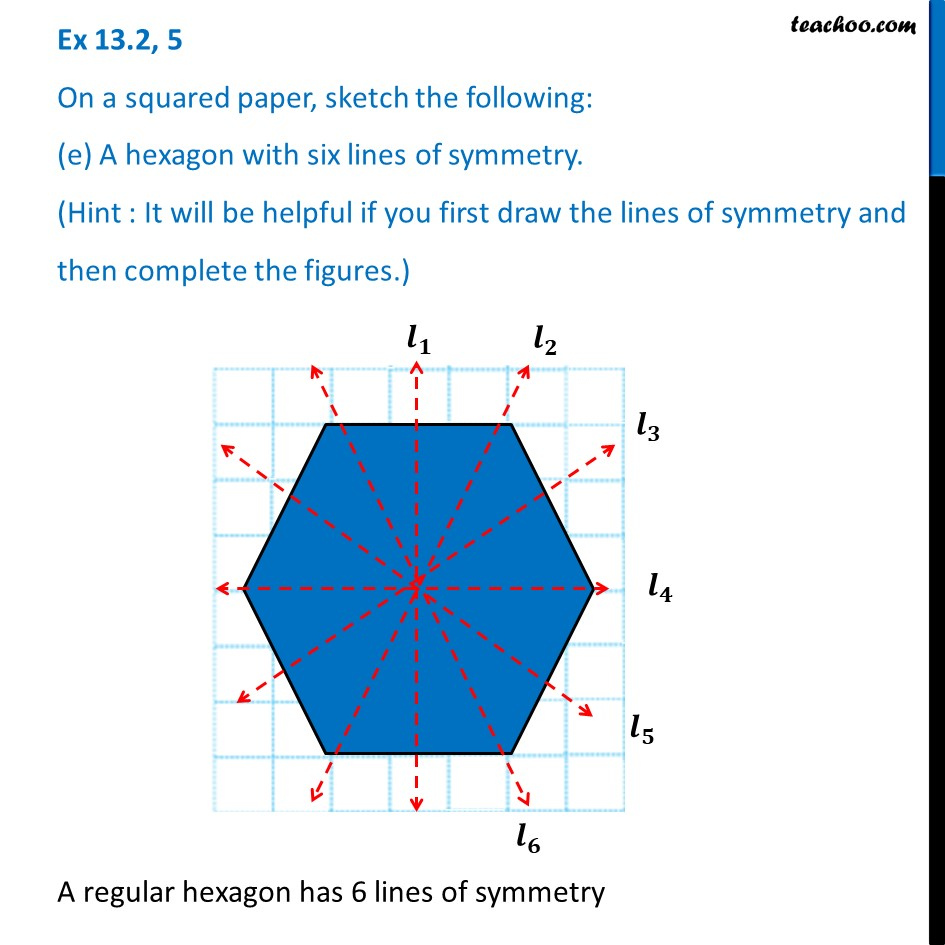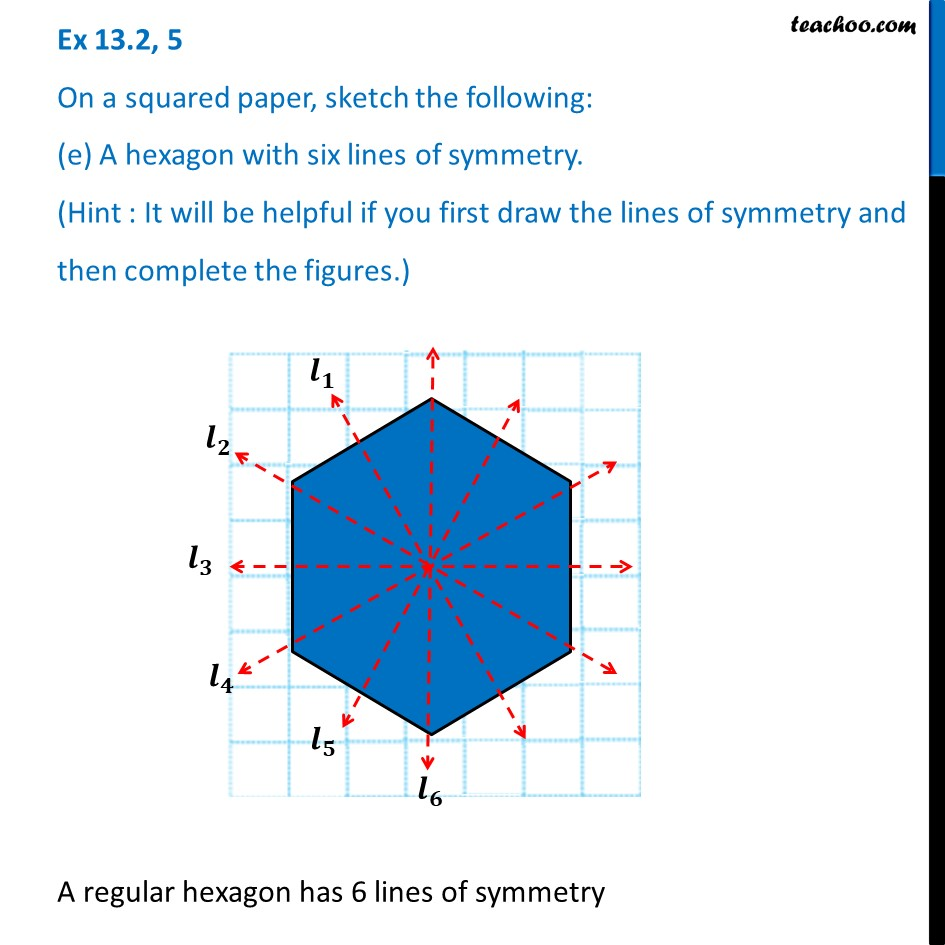Line of Symmetry - regular figures

Symmetry
Serial order wiseLearn in your speed, with individual attention - Teachoo Maths 1-on-1 Class

### Transcript

Question 5 On a squared paper, sketch the following: (e) A hexagon with six lines of symmetry. (Hint : It will be helpful if you first draw the lines of symmetry and then complete the figures.)Question 5 On a squared paper, sketch the following: (e) A hexagon with six lines of symmetry. (Hint : It will be helpful if you first draw the lines of symmetry and then complete the figures.)A regular hexagon has 6 lines of symmetry